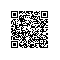# 循环神经网络

## 教程文件

ptb_word_lm.py 在 PTB 数据集上训练一个语言模型.
reader.py 读取数据集.

## 模型

### LSTM

lstm = rnn_cell.BasicLSTMCell(lstm_size)
# 初始化 LSTM 存储状态.
state = tf.zeros([batch_size, lstm.state_size])

loss = 0.0
for current_batch_of_words in words_in_dataset:
# 每次处理一批词语后更新状态值.
output, state = lstm(current_batch_of_words, state)

# LSTM 输出可用于产生下一个词语的预测
logits = tf.matmul(output, softmax_w) + softmax_b
probabilities = tf.nn.softmax(logits)
loss += loss_function(probabilities, target_words)


### 截断反向传播

# 一次给定的迭代中的输入占位符.
words = tf.placeholder(tf.int32, [batch_size, num_steps])

lstm = rnn_cell.BasicLSTMCell(lstm_size)
# 初始化 LSTM 存储状态.
initial_state = state = tf.zeros([batch_size, lstm.state_size])

for i in range(len(num_steps)):
# 每处理一批词语后更新状态值.
output, state = lstm(words[:, i], state)

# 其余的代码.
# ...

final_state = state


# 一个 numpy 数组，保存每一批词语之后的 LSTM 状态.
numpy_state = initial_state.eval()
total_loss = 0.0
for current_batch_of_words in words_in_dataset:
numpy_state, current_loss = session.run([final_state, loss],
# 通过上一次迭代结果初始化 LSTM 状态.
feed_dict={initial_state: numpy_state, words: current_batch_of_words})
total_loss += current_loss


### 输入

# embedding_matrix 张量的形状是： [vocabulary_size, embedding_size]
word_embeddings = tf.nn.embedding_lookup(embedding_matrix, word_ids)


### 损失函数### 多个 LSTM 层堆叠

lstm = rnn_cell.BasicLSTMCell(lstm_size)
stacked_lstm = rnn_cell.MultiRNNCell([lstm] * number_of_layers)

initial_state = state = stacked_lstm.zero_state(batch_size, tf.float32)
for i in range(len(num_steps)):
# 每次处理一批词语后更新状态值.
output, state = stacked_lstm(words[:, i], state)

# 其余的代码.
# ...

final_state = state


## 编译并运行代码

bazel build -c opt tensorflow/models/rnn/ptb:ptb_word_lm


bazel build -c opt --config=cuda tensorflow/models/rnn/ptb:ptb_word_lm


bazel-bin/tensorflow/models/rnn/ptb/ptb_word_lm \
--data_path=/tmp/simple-examples/data/ --alsologtostderr --model small


## 除此之外？

• 随时间降低学习率,
• LSTM 层间 dropout.使用钉钉扫一扫加入圈子
+ 订阅